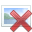# About the angle between force and displacment, W=F.d cosƟ

• Sculter

#### Sculter

HW Template missing as it was moved from another forum
W=F.d cos Ɵ, in a problem Ɵ=30, but in the solution he used Ɵ=60, and vice versa in other problems, can anyone explain why?

Last edited by a moderator:
Is θ the angle between F and d, or is it something else? Please post the full problem so we know what you're talking about.

Is θ the angle between F and d, or is it something else? Please post the full problem so we know what you're talking about.
problem like:

*calculate the force acting on an object if the work done to move it a distance 50m equal 2500J and if the angle between the force and the perpendicular to motion is 30
the answer: w=fd cosθ , F=2500/50xcos60 = 100N

*a man of mass 70kg. goes upstaris of lenth 50m. calculate the work done if g=10m/s2 http://im88.gulfup.com/rmDnTK.png [Broken]
-answer W=Fd cosθ, 70x10x50 x cos30

-problem with no changing in angles-

*a force of 100N acts on an object and moves it a distance 2.5m, calculate the work by the force if the force is inclined by an angle 60 on the direction of motion

----------

i don't know why he changes angles and when, i think I'm missing something.

Last edited by a moderator:
*calculate the force acting on an object if the work done to move it a distance 50m equal 2500J and if the angle between the force and the perpendicular to motion is 30
the answer: w=fd cosθ , F=2500/50xcos60 = 100N
Note that the angle given is not between F and d, but between F and the perpendicular to d. It's up to you to figure out the correct angle!

*a man of mass 70kg. goes upstaris of lenth 50m. calculate the work done if g=10m/s2-answer W=Fd cosθ, 70x10x50 x cos30
Again, the angle given is the angle of the stairs with the horizontal. What you need is the angle between the force (gravity) and the displacement.

-problem with no changing in angles-

*a force of 100N acts on an object and moves it a distance 2.5m, calculate the work by the force if the force is inclined by an angle 60 on the direction of motion
Here they just give you the required angle, so you can just plug it in.

i don't know why he changes angles and when, i think I'm missing something.
To use the work formula you need the angle between the force and the displacement. Sometimes you'll be given that angle directly; other times you'll have to figure out the correct angle to use.

oh, great now i know why, i didn't realize it, thank you
but how do i find the angle?

oh, great now i know why, i didn't realize it, thank you
You are welcome.

but how do i find the angle?
Start by drawing yourself a diagram. And use the properties of right triangles. (For example, the two angles of a right triangle are complementary. They add to 90 degrees.)

Thanky you very much!